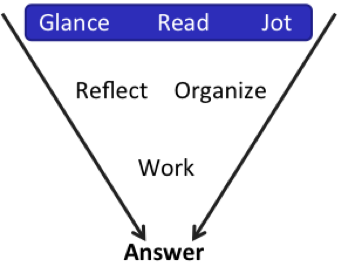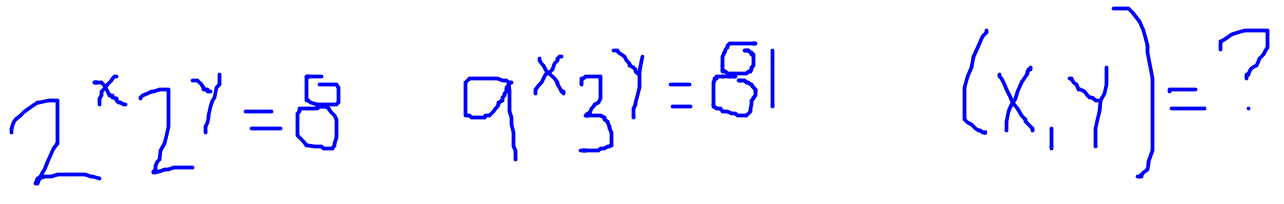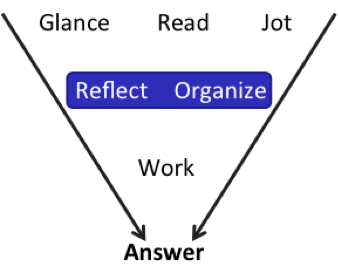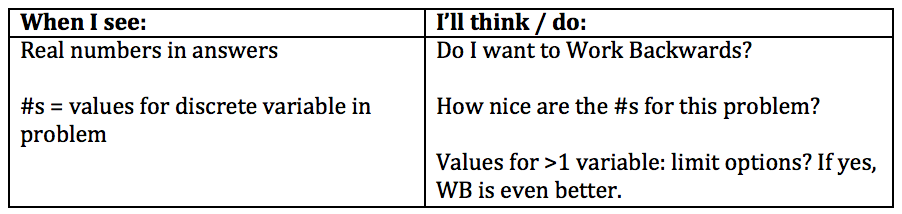# Know the GMAT Code: Working Backwards:

by on July 21st, 2017Do you know how to Work Backwards on Problem Solving (PS) problems? More important, do you know when to work backwards—and when not to? To get a really high score on this test, you’ve got to Know the Code in order to get through the questions efficiently.

I’ve got two problems for you to try from the GMATPrep® free question set. On one, working backwards is a great option. On the other…not so much. If you think you already know this strategy, test out your skills by trying both problems and articulating (out loud, so you know that you really know it!) how to know where you can work backwards and where you shouldn’t try.

If you don’t already know how to work backwards, just go ahead and try both problems however you’d like and then we’ll learn all about this strategy.

Ready? Set your timer for 4 minutes and … Go!

“*Ifand, then“(A) (1, 2)

“(B) (2, 1)

“(C) (1, 1)

“(D) (2, 2)

“(E) (1, 3)”

Ifand, what is the value of?

“(A) -14

“(B)  -2

“(C) 3

“(D) 6

“(E) 18”

Okay, what do you think? Don’t just keep reading. Take a stand now—even if you’re not sure, just guess. (That goes for your actual answers and what you think re: when to work backwards.) Making yourself take a guess invests you more in the outcome—and helps you to better retain what you’re about to learn.

Let’s do this!We’re going to do the first one first. Glance at the problem. PS. Exponents. Glance at the answers … pairs? Is this like coordinate plane or something?

Read. No, it’s not actually geometry—it’s just writing the x and y answers this way. Jot down the given equations.Note that I didn’t repeat the parentheses in the equations when I jotted this down because I’m confident that I won’t make a mistake by removing them. (Though I could make a different mistake!) If you aren’t confident about that, then keep using the parentheses.

All right, let’s think about what we’ve got here.Variables in the exponents. I can solve algebraically by getting the bases on each side to be equal and then dropping the bases and setting the exponents equal to each other. Anything else?

Glance at those answers again. There’s something pretty awesome about them.

First, the answers actually give you the possible values for the only two variables in this problem,and. This is the classic sign that you can work backwards, if you want to: The answer choices give you the actual value for at least one discrete variable in the problem.

But, in this case, it gets even better! In order to work backwards, you have to have that first criterion (actual value for one discrete variable), but there are additional criteria that make the problem easier to do this way. First, the values should be “nice” numbers—they’re not too hard to plug back into the problem. In this case, the possibilities are 1, 2, and 3 … as nice as it gets.Second, tell me what the possible values forare.

Check it out! Although there are 5 answer choices, there are only 2 distinct possibilities for: 1 or 2. Just try them both and you’ll be done in about 1 minute.For the first equation, when,, which matches answer (A). Is that actually the correct answer?

Try the numbers in the second equation to see. If they work, answer (A) is correct. If they don’t, thenmust equal 2, and you’ll have to plug into one of the two equations to find out whatequals.CORRECT!

The pairing (1, 2) does work for the second equation, so the correct answer is (A).

You can of course also solve this problem algebraically, and the algebra is not super hard on this one. But given that you only have to try a maximum of two numbers—and that those two numbers are 1 and 2—working backwards should still be a serious consideration.

Since I told you at the beginning that only one problem could be done by working backwards, you now know that you can’t use this technique for the second one. Why? Use what you’ve learned so far to articulate the answer to my question, then join me next time, when we’ll dive into the full solution!

## Key Takeaways for Knowing the Code:

(1) If the answers give you possible values for (at least) one discrete variable in the problem, then you can work backwards. Should you? If the numbers are also “nice” numbers for the problem, then you should seriously consider it.(2) Practice working backwards enough that you learn how to spot other signs that WB is a great strategy for a particular problem. In this case, the paired answers meant that we had to try only 2 (really easy) numbers—doesn’t get a lot better than that. Even if you felt fine doing this one algebraically, that lesson is a great lesson to learn so that you know how to spot this characteristic on harder problems in future.

(3) Turn that knowledge into Know the Code flash cards:* GMATPrep® questions courtesy of the Graduate Management Admissions Council. Usage of this question does not imply endorsement by GMAC.